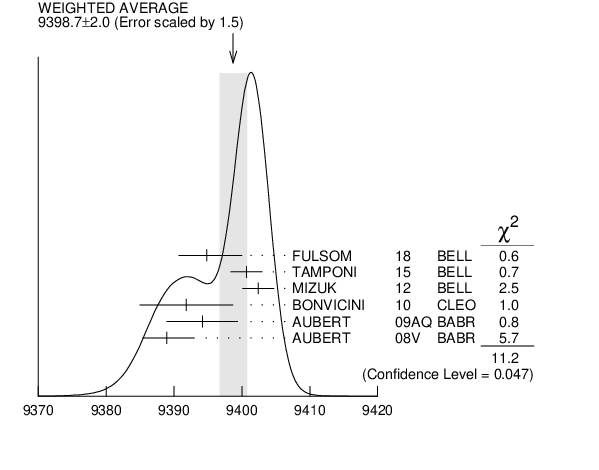# ${{\boldsymbol \eta}_{{b}}{(1S)}}$ MASS INSPIRE search

VALUE (MeV) EVTS DOCUMENT ID TECN  COMMENT
$\bf{ 9398.7 \pm2.0}$ OUR AVERAGE  Error includes scale factor of 1.5.
$9394.8$ ${}^{+2.7}_{-3.1}$ ${}^{+4.5}_{-2.7}$ 29K
 2018
BELL ${{\mathit \Upsilon}{(2S)}}$ $\rightarrow$ ${{\mathit \gamma}}{{\mathit X}}$
$9400.7$ $\pm1.7$ $\pm1.6$ 33.1k
 2015
BELL ${{\mathit e}^{+}}$ ${{\mathit e}^{-}}$ $\rightarrow$ ${{\mathit \gamma}}{{\mathit \eta}}$ + hadrons
$9402.4$ $\pm1.5$ $\pm1.8$ 34k 1
 2012
BELL ${{\mathit e}^{+}}$ ${{\mathit e}^{-}}$ $\rightarrow$ ${{\mathit \gamma}}{{\mathit \pi}^{+}}{{\mathit \pi}^{-}}$ + hadrons
$9391.8$ $\pm6.6$ $\pm2.0$ 2.3k 2
 2010
CLEO ${{\mathit \Upsilon}{(3S)}}$ $\rightarrow$ ${{\mathit \gamma}}{{\mathit X}}$
$9394.2$ ${}^{+4.8}_{-4.9}$ $\pm2.0$ 13k 2
 2009 AQ
BABR ${{\mathit \Upsilon}{(2S)}}$ $\rightarrow$ ${{\mathit \gamma}}{{\mathit X}}$
$9388.9$ ${}^{+3.1}_{-2.3}$ $\pm2.7$ 19k 2
 2008 V
BABR ${{\mathit \Upsilon}{(3S)}}$ $\rightarrow$ ${{\mathit \gamma}}{{\mathit X}}$
• • • We do not use the following data for averages, fits, limits, etc. • • •
$9393.2$ $\pm3.4$ $\pm2.3$ 10 2, 3
 2012
${{\mathit \Upsilon}{(2S)}}$ $\rightarrow$ ${{\mathit \gamma}}$ hadrons
$9300$ $\pm20$ $\pm20$
 2002 D
ALEP $181 - 209$ ${{\mathit e}^{+}}{{\mathit e}^{-}}$
1  With floating width. Not independent of the corresponding mass difference measurement.
2  Assuming ${\Gamma}_{{\mathit \eta}_{{b}}{(1S)}}$ = 10 MeV. Not independent of the corresponding ${{\mathit \gamma}}$ energy or mass difference measurements.
3  Obtained by analyzing CLEO III data but not authored by the CLEO Collaboration.${{\mathit \eta}_{{b}}{(1S)}}$ MASS (MeV)
References:
 FULSOM 2018
PRL 121 232001 Observation of $\Upsilon(2S)\to\gamma \eta_{b}(1S)$ decay
 TAMPONI 2015
PRL 115 142001 First Observation of the Hadronic Transition ${{\mathit \Upsilon}{(4S)}}$ $\rightarrow$ ${{\mathit \eta}}{{\mathit h}_{{b}}{(1P)}}$ and New Measurement of the ${{\mathit h}_{{b}}{(1P)}}$ and ${{\mathit \eta}_{{b}}{(1S)}}$ PParameters
 DOBBS 2012
PRL 109 082001 Observation of the ${{\mathit \eta}_{{b}}{(2S)}}$ Meson in ${{\mathit \Upsilon}{(2S)}}$ $\rightarrow$ ${{\mathit \gamma}}{{\mathit \eta}_{{b}}{(2S)}}$ , ${{\mathit \eta}_{{b}}{(2S)}}$ $\rightarrow$ Hadrons and Confirmation of the ${{\mathit \eta}_{{b}}{(1S)}}$ Meson
 MIZUK 2012
PRL 109 232002 Evidence for the ${{\mathit \eta}_{{b}}{(2S)}}$ and Observation of ${{\mathit h}_{{b}}{(1P)}}$ $\rightarrow$ ${{\mathit \eta}_{{b}}{(1S)}}{{\mathit \gamma}}$ and ${{\mathit h}_{{b}}{(2P)}}$ $\rightarrow$ ${{\mathit \eta}_{{b}}{(1S)}}{{\mathit \gamma}}$
 BONVICINI 2010
PR D81 031104 Measurement of the ${{\mathit \eta}_{{b}}{(1S)}}$ Mass and the Branching Fraction for ${{\mathit \Upsilon}{(3S)}}$ $\rightarrow$ ${{\mathit \gamma}}{{\mathit \eta}_{{b}}{(1S)}}$
 AUBERT 2009AQ
PRL 103 161801 Evidence for the ${{\mathit \eta}_{{b}}{(1S)}}$ Meson in Radiative ${{\mathit \Upsilon}{(2S)}}$ Decay
 AUBERT 2008V
PRL 101 071801 Observation of the Bottomonium Ground State in the Decay ${{\mathit \Upsilon}{(3S)}}$ $\rightarrow$ ${{\mathit \gamma}}{{\mathit \eta}_{{b}}}$
 HEISTER 2002D
PL B530 56 Search for ${{\mathit \gamma}}$ ${{\mathit \gamma}}$ $\rightarrow$ ${{\mathit \eta}_{{b}}}$ in ${{\mathit e}^{+}}{{\mathit e}^{-}}$ Collisions at LEP-2